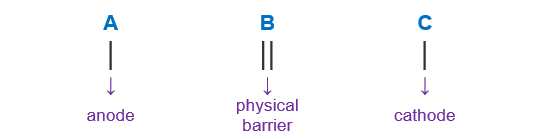# Problem: A student devises an electrochemical cell with the following cell notation:Cr (s) | Cr2+ (aq, 0.02 M) | | Co2+ (aq, 0.9 M) | Co (s)Calculate Δε for this galvanic cell. Show all work and circle and circle your final answer.

###### FREE Expert Solution

For this problem, we’re being asked to calculate for the Ecell with the cell notation:

Cr (s) | Cr2+ (aq, 0.02 M) | | Co2+ (aq, 0.9 M) | Co (s)

To do so, follow these steps:

Step 1. Determine the E°cell and value of n

Step 2. Calculate Ecell

Step 1. When writing a cell notation, we use the following format – “as easy as ABCLoss of Electron is an Oxidation process undergone by the Reducing Agent (LEORA). In the Anode, Oxidation happens (An Ox)

Gain of Electron is a Reduction process undergone by the Oxidizing Agent (GEROA). Reduction happens in the Cathode (Red Cat)

We can determine the anode and cathode by comparing their E° values.

• ↓ E° → oxidation → anode

• ↑ E° → reduction → cathode

96% (472 ratings)###### Problem Details

A student devises an electrochemical cell with the following cell notation:

Cr (s) | Cr2+ (aq, 0.02 M) | | Co2+ (aq, 0.9 M) | Co (s)

Calculate Δε for this galvanic cell. Show all work and circle and circle your final answer.